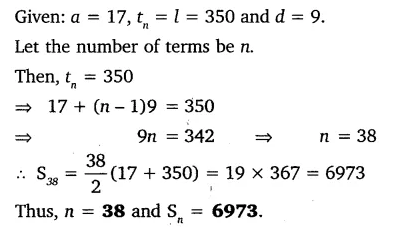# The first and the last terms of an AP are 17 and 350 respectively

The first and the last terms of an AP are 17 and 350 respectively. If the common difference is 9, how many terms are there and what is their sum?